# 武科大流体力学Chapter3.ppt

1 de Jun de 2023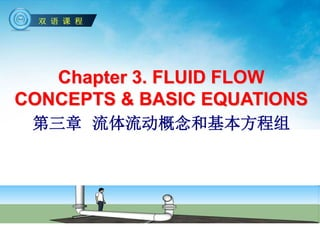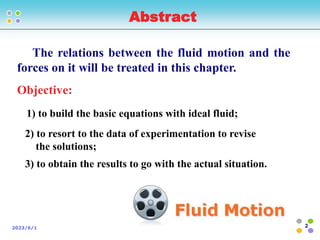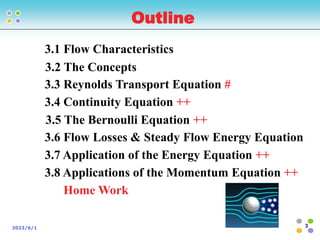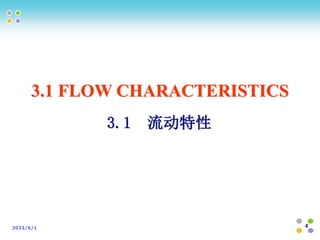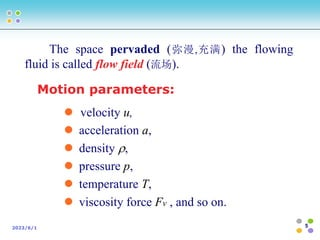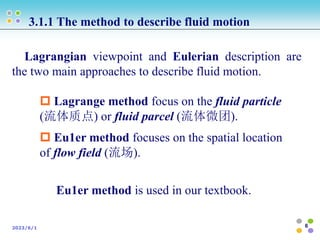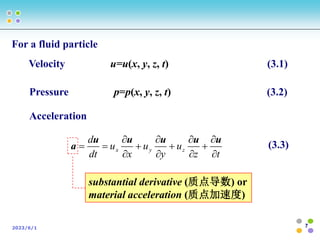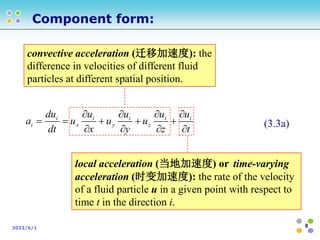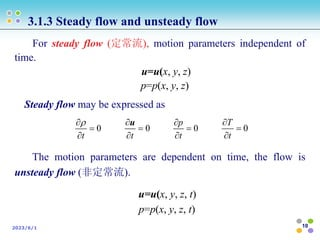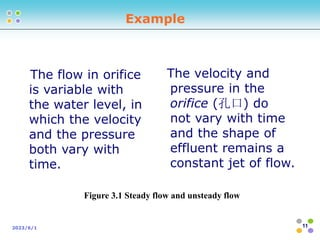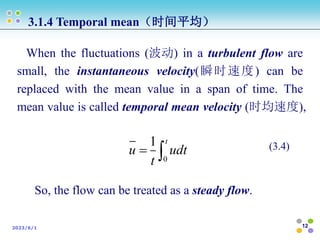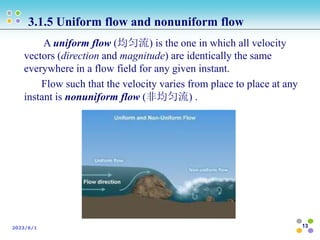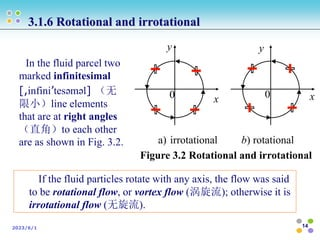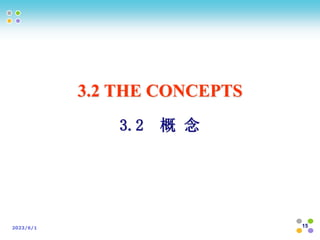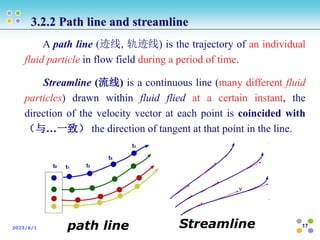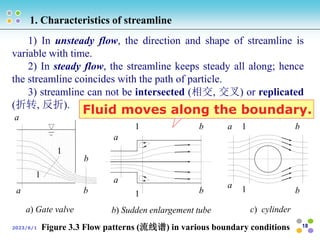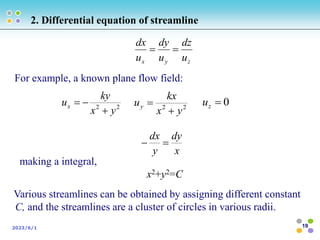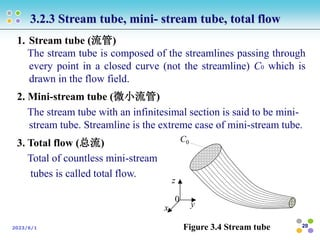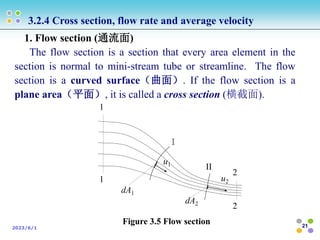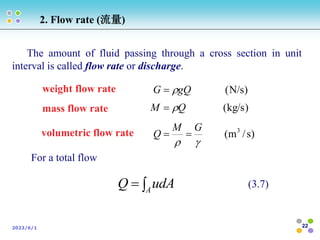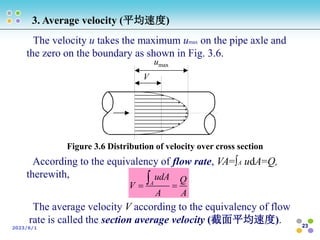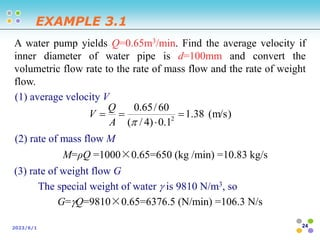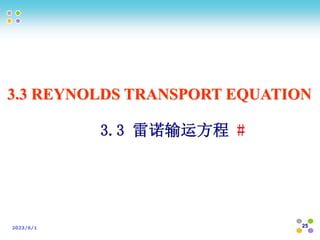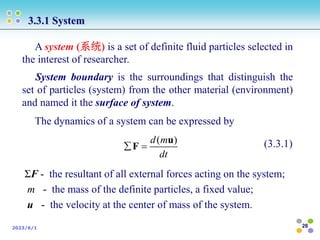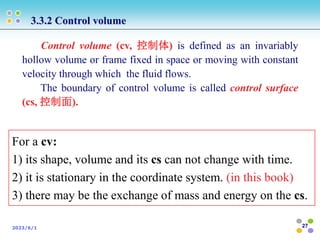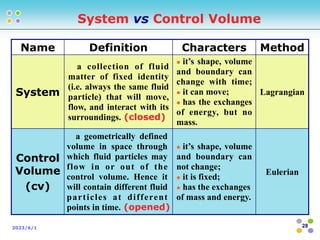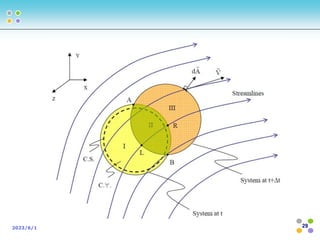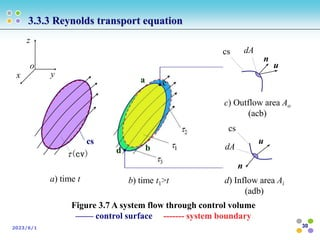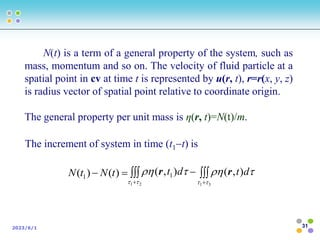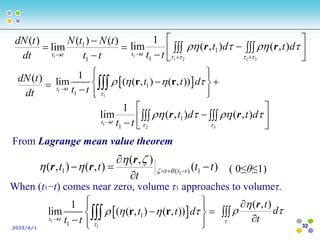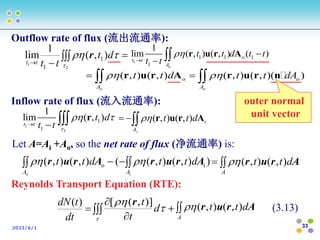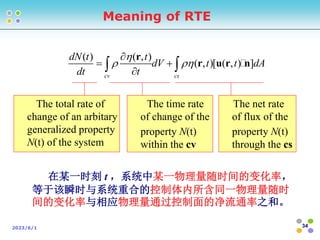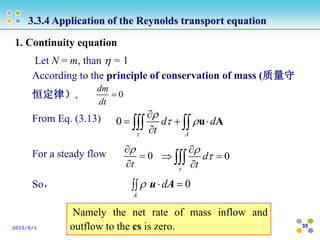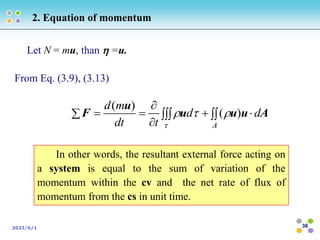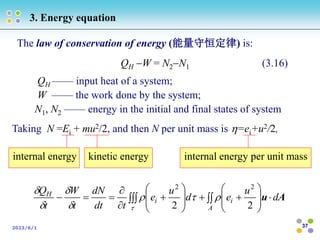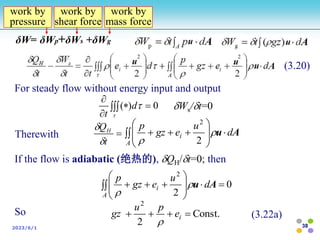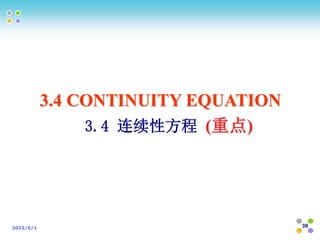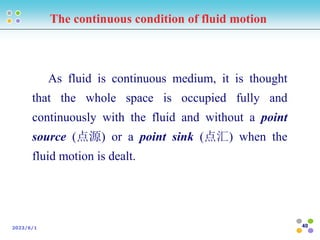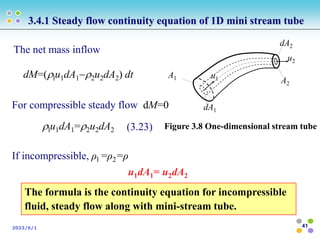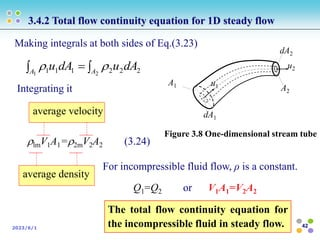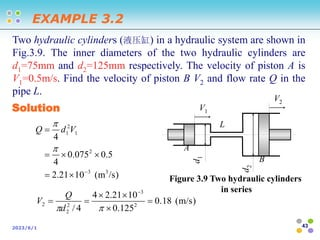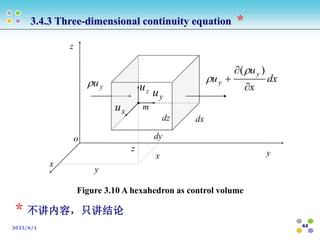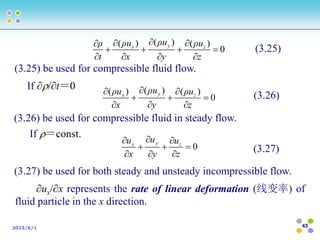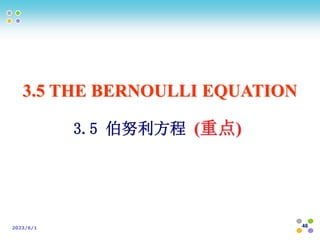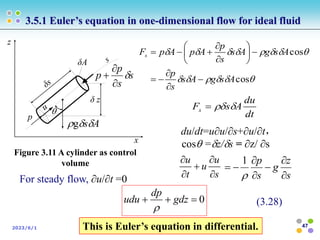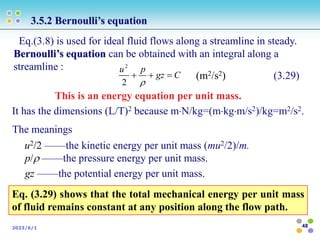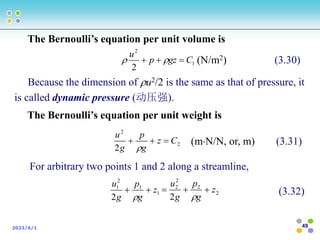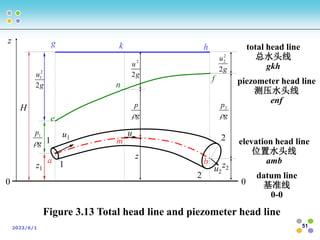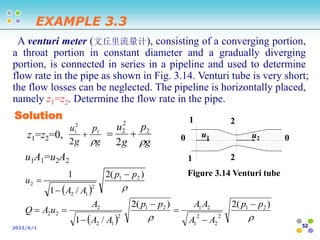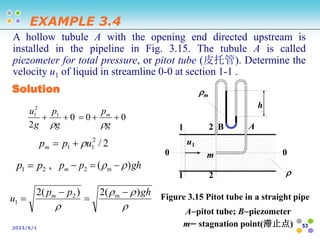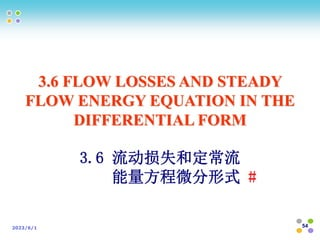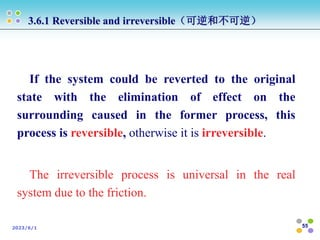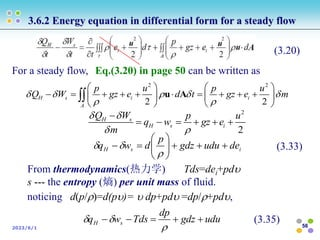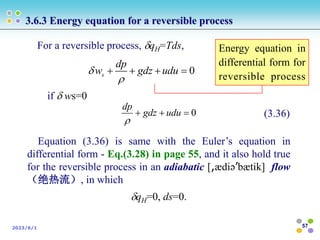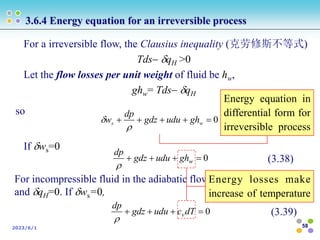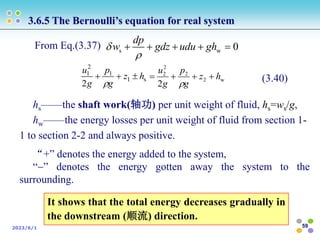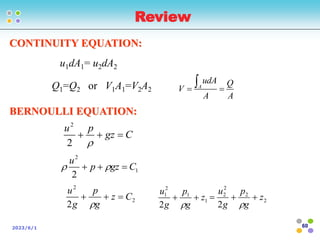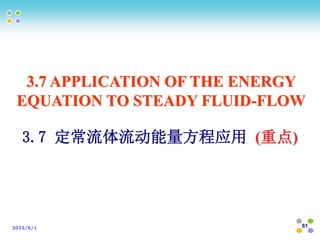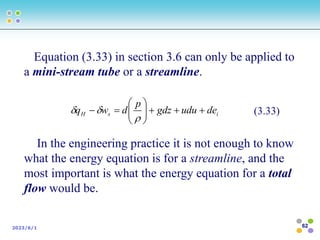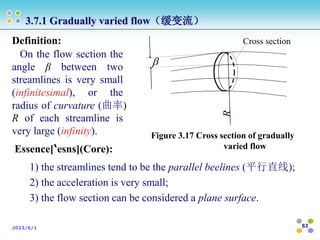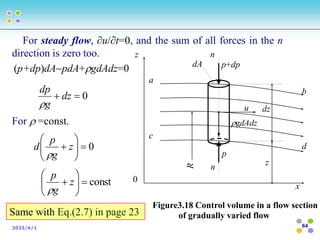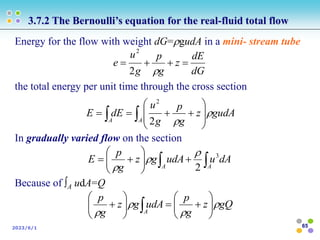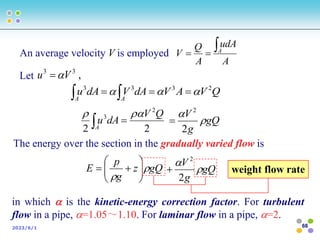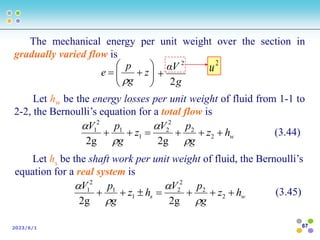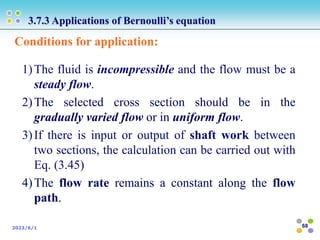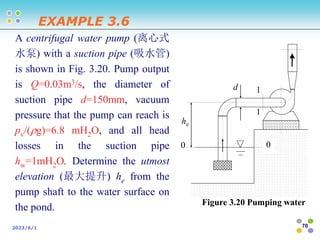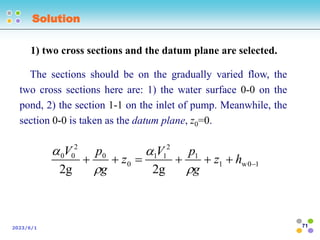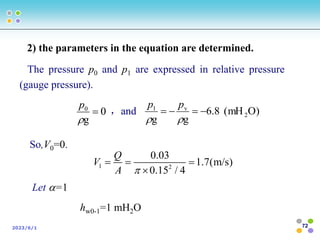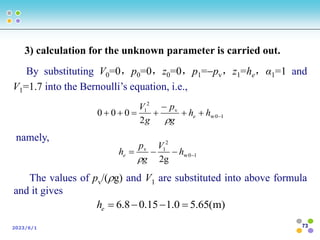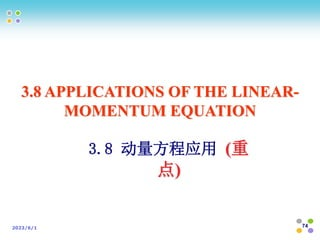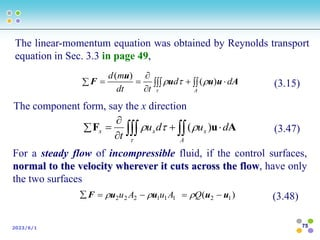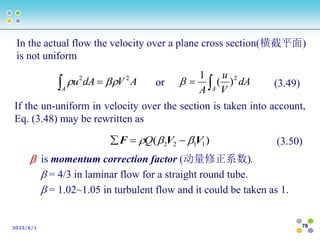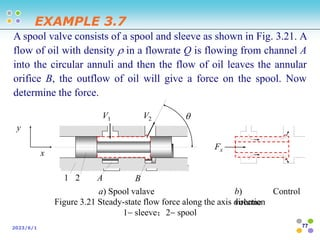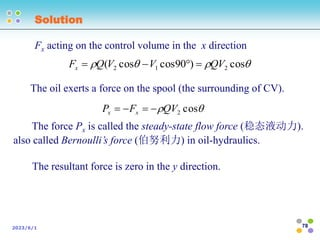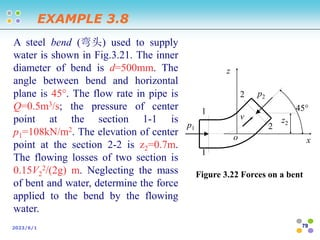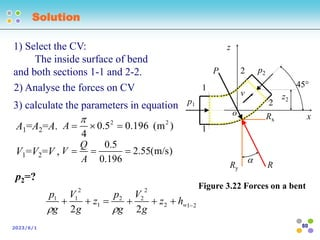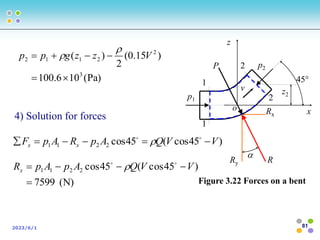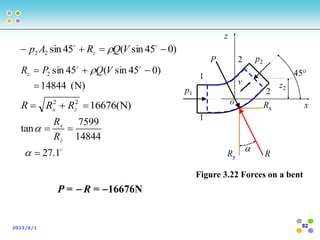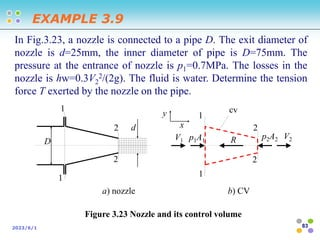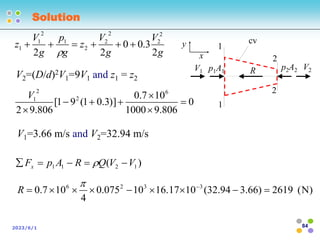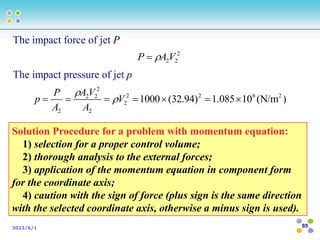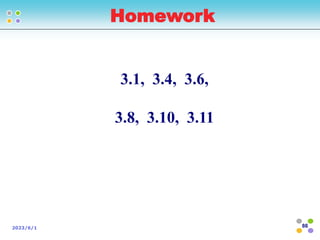1 de 86

### 武科大流体力学Chapter3.ppt

• 1. Chapter 3. FLUID FLOW CONCEPTS & BASIC EQUATIONS 第三章 流体流动概念和基本方程组 双 语 课 程
• 2. 2 2023/6/1 The relations between the fluid motion and the forces on it will be treated in this chapter. Objective: 1) to build the basic equations with ideal fluid; 2) to resort to the data of experimentation to revise the solutions; 3) to obtain the results to go with the actual situation. Abstract
• 3. 3 2023/6/1 3.1 Flow Characteristics 3.2 The Concepts 3.3 Reynolds Transport Equation # 3.4 Continuity Equation ++ 3.5 The Bernoulli Equation ++ 3.6 Flow Losses & Steady Flow Energy Equation 3.7 Application of the Energy Equation ++ 3.8 Applications of the Momentum Equation ++ Home Work Outline
• 5. 5 2023/6/1 The space pervaded (弥漫,充满) the flowing fluid is called flow field (流场).  velocity u,  acceleration a,  density ,  pressure p,  temperature T,  viscosity force Fv , and so on. Motion parameters:
• 6. 6 2023/6/1 3.1.1 The method to describe fluid motion Lagrangian viewpoint and Eulerian description are the two main approaches to describe fluid motion.  Lagrange method focus on the fluid particle (流体质点) or fluid parcel (流体微团).  Eu1er method focuses on the spatial location of flow field (流场). Eu1er method is used in our textbook.
• 7. 7 2023/6/1 Velocity u=u(x, y, z, t) (3.1) Pressure p=p(x, y, z, t) (3.2) Acceleration t z u y u x u dt d z y x              u u u u u a (3.3) For a fluid particle substantial derivative (质点导数) or material acceleration (质点加速度)
• 8. 8 2023/6/1 t u z u u y u u x u u dt du a i i z i y i x i i              (3.3a) convective acceleration (迁移加速度): the difference in velocities of different fluid particles at different spatial position. local acceleration (当地加速度) or time-varying acceleration (时变加速度): the rate of the velocity of a fluid particle u in a given point with respect to time t in the direction i. Component form:
• 9. 9 2023/6/1 3.1.2 Laminar flow and turbulent flow Reynolds (雷诺), a British scientist, showed that there are two basic types of fluid flow: laminar and turbulent flow in 1883. laminar flow (层流): The fluid flows orderly. One layer of fluid particle moves smoothly over another layer. The particles are not mixed with each other. The viscous force meets the Newton’s law of viscosity,v=du/dy. Turbulent flow (紊流, 湍流)： Very complicated and irregular motion trajectories; There is transverse motion as well; The viscous frictions of turbulent flow consist of not only the viscous friction but also the turbulent shear resistance, t=uxuy
• 10. 10 2023/6/1 3.1.3 Steady flow and unsteady flow For steady flow (定常流), motion parameters independent of time. u=u(x, y, z) p=p(x, y, z) Steady flow may be expressed as The motion parameters are dependent on time, the flow is unsteady flow (非定常流). 0    t  0    t u 0    t p 0    t T u=u(x, y, z, t) p=p(x, y, z, t)
• 11. 11 2023/6/1 a) steady flow b) unsteady flow Figure 3.1 Steady flow and unsteady flow 0 0 H water supply overfall(溢流道) orifice(孔口) 0 0 H 3 H 2 H 1 t1 t2 t3 orifice Example The velocity and pressure in the orifice (孔口) do not vary with time and the shape of effluent remains a constant jet of flow. The flow in orifice is variable with the water level, in which the velocity and the pressure both vary with time.
• 12. 12 2023/6/1 When the fluctuations (波动) in a turbulent flow are small, the instantaneous velocity(瞬时速度) can be replaced with the mean value in a span of time. The mean value is called temporal mean velocity (时均速度), 3.1.4 Temporal mean（时间平均） 0 1 t u udt t   (3.4) So, the flow can be treated as a steady flow.
• 13. 13 2023/6/1 A uniform flow (均匀流) is the one in which all velocity vectors (direction and magnitude) are identically the same everywhere in a flow field for any given instant. Flow such that the velocity varies from place to place at any instant is nonuniform flow (非均匀流) . 3.1.5 Uniform flow and nonuniform flow
• 14. 14 2023/6/1 3.1.6 Rotational and irrotational a) irrotational b) rotational Figure 3.2 Rotational and irrotational In the fluid parcel two marked infinitesimal [,infini’tesəməl] （无 限小）line elements that are at right angles （直角）to each other are as shown in Fig. 3.2. y x y x 0 0 If the fluid particles rotate with any axis, the flow was said to be rotational flow, or vortex flow (涡旋流); otherwise it is irrotational flow (无旋流).
• 16. 16 2023/6/1 One dimension——all main variables in the flow field can be completely specified by a single coordinate if the variation of flow parameters transverse to the mainstream direction can be neglected. Flow in parallel planes is two-dimensional because the motion of fluid particle normal to these planes is restricted for the existence of the planes. Three-dimensional flow is the most general flow. 3.2.1 Flow dimensionality （流的维度）
• 17. 17 2023/6/1 A path line (迹线, 轨迹线) is the trajectory of an individual fluid particle in flow field during a period of time. Streamline (流线) is a continuous line (many different fluid particles) drawn within fluid flied at a certain instant, the direction of the velocity vector at each point is coincided with （与…一致） the direction of tangent at that point in the line. 3.2.2 Path line and streamline path line Streamline
• 18. 18 2023/6/1 1) In unsteady flow, the direction and shape of streamline is variable with time. 2) In steady flow, the streamline keeps steady all along; hence the streamline coincides with the path of particle. 3) streamline can not be intersected (相交, 交叉) or replicated (折转, 反折). 1. Characteristics of streamline a a b b 1 1 a a b b 1 1 a a b b 1 1 a) Gate valve b) Sudden enlargement tube c) cylinder Figure 3.3 Flow patterns (流线谱) in various boundary conditions Fluid moves along the boundary.
• 19. 19 2023/6/1 z y x u dz u dy u dx   For example, a known plane flow field: 2 2 y x ky ux    2 2 y x kx uy   0  z u x dy y dx   making a integral, x2+y2=C 2. Differential equation of streamline Various streamlines can be obtained by assigning different constant C, and the streamlines are a cluster of circles in various radii.
• 20. 20 2023/6/1 1. Stream tube (流管) The stream tube is composed of the streamlines passing through every point in a closed curve (not the streamline) C0 which is drawn in the flow field. 2. Mini-stream tube (微小流管) The stream tube with an infinitesimal section is said to be mini- stream tube. Streamline is the extreme case of mini-stream tube. 3. Total flow (总流) Total of countless mini-stream tubes is called total flow. 3.2.3 Stream tube, mini- stream tube, total flow Figure 3.4 Stream tube C0 y z 0 x
• 21. 21 2023/6/1 3.2.4 Cross section, flow rate and average velocity 1. Flow section (通流面) The flow section is a section that every area element in the section is normal to mini-stream tube or streamline. The flow section is a curved surface（曲面）. If the flow section is a plane area（平面）, it is called a cross section (横截面). 1 1 2 2 I II u1 u2 dA1 dA2 Figure 3.5 Flow section
• 22. 22 2023/6/1 The amount of fluid passing through a cross section in unit interval is called flow rate or discharge. ) s / m ( ) kg/s ( ) N/s ( 3     G M Q Q M gQ G     weight flow rate volumetric flow rate mass flow rate   A udA Q (3.7) For a total flow 2. Flow rate (流量)
• 23. 23 2023/6/1 3. Average velocity (平均速度) umax V Figure 3.6 Distribution of velocity over cross section A udA Q V A A    The velocity u takes the maximum umax on the pipe axle and the zero on the boundary as shown in Fig. 3.6. The average velocity V according to the equivalency of flow rate is called the section average velocity (截面平均速度). According to the equivalency of flow rate, VA=∫A udA=Q, therewith,
• 24. 24 2023/6/1 A water pump yields Q=0.65m3/min. Find the average velocity if inner diameter of water pipe is d=100mm and convert the volumetric flow rate to the rate of mass flow and the rate of weight flow. (1) average velocity V ) m/s ( 38 . 1 1 . 0 ) 4 / ( 60 / 65 . 0 2      A Q V (2) rate of mass flow M M=ρQ =1000×0.65=650 (kg /min) =10.83 kg/s (3) rate of weight flow G The special weight of water  is 9810 N/m3, so G=Q=9810×0.65=6376.5 (N/min) =106.3 N/s EXAMPLE 3.1
• 25. 25 2023/6/1 3.3 REYNOLDS TRANSPORT EQUATION 3.3 雷诺输运方程 #
• 26. 26 2023/6/1 3.3.1 System A system (系统) is a set of definite fluid particles selected in the interest of researcher. System boundary is the surroundings that distinguish the set of particles (system) from the other material (environment) and named it the surface of system. ( ) d m dt   u F (3.3.1) The dynamics of a system can be expressed by ΣF - the resultant of all external forces acting on the system; m - the mass of the definite particles, a fixed value; u - the velocity at the center of mass of the system.
• 27. 27 2023/6/1 3.3.2 Control volume Control volume (cv, 控制体) is defined as an invariably hollow volume or frame fixed in space or moving with constant velocity through which the fluid flows. The boundary of control volume is called control surface (cs, 控制面). For a cv: 1) its shape, volume and its cs can not change with time. 2) it is stationary in the coordinate system. (in this book) 3) there may be the exchange of mass and energy on the cs.
• 28. 28 2023/6/1 Name Definition Characters Method System a collection of fluid matter of fixed identity (i.e. always the same fluid particle) that will move, flow, and interact with its surroundings. (closed)  it’s shape, volume and boundary can change with time;  it can move;  has the exchanges of energy, but no mass. Lagrangian Control Volume (cv) a geometrically defined volume in space through which fluid particles may flow in or out of the control volume. Hence it will contain different fluid particles at different points in time. (opened)  it’s shape, volume and boundary can not change;  it is fixed;  has the exchanges of mass and energy. Eulerian System vs Control Volume
• 30. 30 2023/6/1 3.3.3 Reynolds transport equation Figure 3.7 A system flow through control volume —— control surface ------- system boundary (cv) a) time t y x z o b) time t1>t u n cs dA d) Inflow area Ai (adb) u dA n cs c) Outflow area Ao (acb) cs a b c d
• 31. 31 2023/6/1 N(t) is a term of a general property of the system, such as mass, momentum and so on. The velocity of fluid particle at a spatial point in cv at time t is represented by u(r, t), r=r(x, y, z) is radius vector of spatial point relative to coordinate origin. The general property per unit mass is η(r, t)=N(t)/m. The increment of system in time (t1t) is   ) ( ) ( 1 t N t N   2 1 ) , ( 1     d t r    3 1 ) , (     d t r
• 32. 32 2023/6/1  dt t dN ) (   1 1 1 1 1 lim ( ( , ) ( , )) t t t t d t t                     r r            2 3 1 ) , ( ) , ( 1 lim 1 1       d t d t t t t t r r From Lagrange mean value theorem ) ( ) , ( ) , ( ) , ( 1 ) ( 1 1 t t t t t t t t               r r r ( 0≤θ≤1)        d t t) , (r   1 1 1 1 1 lim ( ( , ) ( , )) t t t t d t t                     r r      t t t N t N dt t dN t t 1 1 ) ( ) ( lim ) ( 1              2 1 3 1 1 ) , ( ) , ( 1 lim 1 1         d t d t t t t t r r When (t1−t) comes near zero, volume τ1 approaches to volumeτ.
• 33. 33 2023/6/1     2 1 ) , ( 1 lim 1 1    d t t t t t r 1 1 1 1 1 1 lim ( , ) ( , ) ( ) o o t t A t t d t t t t      r u r A ( , ) ( , ) ( , ) ( , )( ) o o o o A A t t d t t dA       r u r A r u r n 1 3 1 1 1 lim ( , ) t t t d t t       r ( , ) ( , ) i i A t t d    r u r A Let A=Ai +Ao, so the net rate of flux (净流通率) is:   o ) , ( ) , ( A r u r d t t o A  ) ) , ( ) , ( ( i A r u r d t t i A    A r u r d t t A ) , ( ) , (     dt t dN ) (        d t t)] , ( [ r A r u r d t t A ) , ( ) , (  (3.13) outer normal unit vector Outflow rate of flux (流出流通率): Inflow rate of flux (流入流通率): Reynolds Transport Equation (RTE):
• 34. 34 2023/6/1 ( ) ( , ) ( , )[ ( , ) ] cv cs dN t t dV t t dA dt t          r r u r n The total rate of change of an arbitary generalized property N(t) of the system The time rate of change of the property N(t) within the cv The net rate of flux of the property N(t) through the cs 在某一时刻 t ，系统中某一物理量随时间的变化率， 等于该瞬时与系统重合的控制体内所含同一物理量随时 间的变化率与相应物理量通过控制面的净流通率之和。 Meaning of RTE
• 35. 35 2023/6/1 3.3.4 Application of the Reynolds transport equation 1. Continuity equation Let N = m, than  = 1 According to the principle of conservation of mass (质量守 恒定律）, 0  dt dm From Eq. (3.13) 0 A d d t            u A For a steady flow 0 t     0    A dA u  So， 0 d t         Namely the net rate of mass inflow and outflow to the cs is zero.
• 36. 36 2023/6/1 2. Equation of momentum Let N = mu, than  =u. From Eq. (3.9), (3.13)          A d d t dt m d A u u u u F ) ( ) (     In other words, the resultant external force acting on a system is equal to the sum of variation of the momentum within the cv and the net rate of flux of momentum from the cs in unit time.
• 37. 37 2023/6/1 3. Energy equation QH —— input heat of a system; W —— the work done by the system; N1, N2 —— energy in the initial and final states of system QH W = N2N1 (3.16) Taking N =Ei + mu2/2, and then N per unit mass is =ei+u2/2,                            A i i H d u e d u e t dt dN t W t Q A u 2 2 2 2         The law of conservation of energy (能量守恒定律) is: internal energy kinetic energy internal energy per unit mass
• 38. 38 2023/6/1 For steady flow without energy input and output        0 ) ( d t Ws/t=0  t QH                A i d u e gz p A u   2 2 Therewith If the flow is adiabatic (绝热的), QH/t=0; then 0 2 2               A i d u e gz p A u   So Const. 2 2     i e p u gz  (3.22a) δW= δWp+δWs +δWg work by pressure work by shear force work by mass force (3.20)
• 39. 39 2023/6/1 3.4 CONTINUITY EQUATION 3.4 连续性方程 (重点)
• 40. 40 2023/6/1 As fluid is continuous medium, it is thought that the whole space is occupied fully and continuously with the fluid and without a point source (点源) or a point sink (点汇) when the fluid motion is dealt. The continuous condition of fluid motion
• 41. 41 2023/6/1 3.4.1 Steady flow continuity equation of 1D mini stream tube dA1 u1 dA2 u2 A1 A2 Figure 3.8 One-dimensional stream tube The net mass inflow dM=(lu1dA12u2dA2) dt For compressible steady flow dM=0 lu1dA1=2u2dA2 If incompressible, ρl =ρ2=ρ u1dA1= u2dA2 The formula is the continuity equation for incompressible fluid, steady flow along with mini-stream tube. (3.23)
• 42. 42 2023/6/1 3.4.2 Total flow continuity equation for 1D steady flow    2 1 2 2 2 1 1 1 A A dA u dA u   lmV1A1=2mV2A2 (3.24) For incompressible fluid flow, ρ is a constant. Q1=Q2 or V1A1=V2A2 dA1 u1 dA2 u2 A1 A2 Figure 3.8 One-dimensional stream tube Making integrals at both sides of Eq.(3.23) Integrating it average density average velocity The total flow continuity equation for the incompressible fluid in steady flow.
• 43. 43 2023/6/1 V1 V2 d 1 A B L d 2 Figure 3.9 Two hydraulic cylinders in series Two hydraulic cylinders (液压缸) in a hydraulic system are shown in Fig.3.9. The inner diameters of the two hydraulic cylinders are d1=75mm and d2=125mm respectively. The velocity of piston A is V1=0.5m/s. Find the velocity of piston B V2 and flow rate Q in the pipe L. /s) (m 10 21 . 2 5 . 0 075 . 0 4 4 3 3 2 1 2 1          V d Q Solution (m/s) 0.18 125 . 0 10 21 . 2 4 4 / 2 3 2 2 2         d Q V EXAMPLE 3.2
• 44. 44 2023/6/1 3.4.3 Three-dimensional continuity equation * * 不讲内容，只讲结论 y z o y u  dx x u u y y    ) (  y x x z m dx dy dz y u x u z u Figure 3.10 A hexahedron as control volume
• 45. 45 2023/6/1 0 ) ( ) ( ) (             z ρu y ρu x ρu t ρ z y x (3.25) If /t＝0 0 ) ( ) ( ) (          z ρu y ρu x ρu z y x 0          z u y u x u z y x If ＝const. (3.27) be used for both steady and unsteady incompressible flow. ux/x represents the rate of linear deformation (线变率) of fluid particle in the x direction. (3.26) (3.27) (3.26) be used for compressible fluid in steady flow. (3.25) be used for compressible fluid flow.
• 46. 46 2023/6/1 3.5 THE BERNOULLI EQUATION 3.5 伯努利方程 (重点)
• 47. 47 2023/6/1 3.5.1 Euler’s equation in one-dimensional flow for ideal fluid x z p s s p p     δA A s  g  Figure 3.11 A cylinder as control volume δ z               cos cos A s g A s s p A s g A s s p A p A p Fs                  dt du A s Fs    du/dt=uu/s+u/t， cos =z/s = z/ s s u u t u      s z g s p         1 For steady flow, u/t =0 0    gdz dp udu  (3.28) This is Euler’s equation in differential.
• 48. 48 2023/6/1 3.5.2 Bernoulli’s equation Eq.(3.8) is used for ideal fluid flows along a streamline in steady. Bernoulli’s equation can be obtained with an integral along a streamline : C gz p u     2 2 (m2/s2) (3.29) This is an energy equation per unit mass. It has the dimensions (L/T)2 because mN/kg=(mkgm/s2)/kg=m2/s2. The meanings u2/2 ——the kinetic energy per unit mass (mu2/2)/m. p/ ——the pressure energy per unit mass. gz ——the potential energy per unit mass. Eq. (3.29) shows that the total mechanical energy per unit mass of fluid remains constant at any position along the flow path.
• 49. 49 2023/6/1 The Bernoulli’s equation per unit volume is (N/m2) (3.30) 1 2 2 C gz p u      Because the dimension of u2/2 is the same as that of pressure, it is called dynamic pressure (动压强). The Bernoulli’s equation per unit weight is 2 2 2 C z g p g u     (mN/N, or, m) (3.31) For arbitrary two points 1 and 2 along a streamline, 1 1 2 1 2 z g p g u    2 2 2 2 2 z g p g u     (3.32)
• 50. 50 2023/6/1 u h u Figure 3.12 Velocity head hu 3.5.3 Geometry expression of Bernoulli’s equation the Bernoulli’s equation per unit weight is 2 2 2 C z g p g u     (mN/N, or, m) (3.31) Dimension: z —— L，called the elevation head (位置水头). p/(g)——MLT2L2/(ML3LT2)=L, called the pressure head (压力水头). u2/(2g) ——L2T2/(LT2)=L, called the velocity head (速度水头), denoted as hu The sum of them is called the total head (总水头) , denoted as H.
• 51. 51 2023/6/1 Figure 3.13 Total head line and piezometer head line z1 z 0 0 z2 g p  1 g p  g p  2 g u 2 2 g u 2 2 1 g u 2 2 2 z a b g h e f m u1 u u2 2 2 1 1 n k total head line 总水头线 gkh piezometer head line 测压水头线 enf elevation head line 位置水头线 amb datum line 基准线 0-0 H
• 52. 52 2023/6/1 A venturi meter (文丘里流量计), consisting of a converging portion, a throat portion in constant diameter and a gradually diverging portion, is connected in series in a pipeline and used to determine flow rate in the pipe as shown in Fig. 3.14. Venturi tube is very short; the flow losses can be neglected. The pipeline is horizontally placed, namely z1=z2. Determine the flow rate in the pipe. Solution 1 1 2 2 u1 u2 0 0 Figure 3.14 Venturi tube z1=z2=0, g p g u  1 2 1 2  g p g u  2 2 2 2   u1A1=u2A2    ) ( 2 / 1 1 2 1 2 1 2 2 p p A A u        ) ( 2 ) ( 2 / 1 2 1 2 2 2 1 2 1 2 1 2 1 2 2 2 2 p p A A A A p p A A A u A Q        EXAMPLE 3.3
• 53. 53 2023/6/1 A hollow tubule A with the opening end directed upstream is installed in the pipeline in Fig. 3.15. The tubule A is called piezometer for total pressure, or pitot tube (皮托管). Determine the velocity u1 of liquid in streamline 0-0 at section 1-1 . Solution 0 2 1 2 1   g p g u  0 0    g pm  2 / 2 1 1 u p pm        gh p p u m ) ( 2 ) ( 2 m 2 1     gh p pm ) ( m 2      0 0 1 1 h 2 B 2 m u1 A  m Figure 3.15 Pitot tube in a straight pipe Apitot tube; Bpiezometer m stagnation point(滞止点) EXAMPLE 3.4 , 2 1 p p 
• 54. 54 2023/6/1 3.6 FLOW LOSSES AND STEADY FLOW ENERGY EQUATION IN THE DIFFERENTIAL FORM 3.6 流动损失和定常流 能量方程微分形式 #
• 55. 55 2023/6/1 3.6.1 Reversible and irreversible（可逆和不可逆） If the system could be reverted to the original state with the elimination of effect on the surrounding caused in the former process, this process is reversible, otherwise it is irreversible. The irreversible process is universal in the real system due to the friction.
• 56. 56 2023/6/1 3.6.2 Energy equation in differential form for a steady flow i s H de udu gdz p d w q                 From thermodynamics(热力学) Tds=dei+pd s --- the entropy (熵) per unit mass of fluid. noticing d(p/)=d(p)=  dp+pd =dp/+pd, udu gdz dp Tds w q s H         (3.35) (3.20) For a steady flow, (3.33) 2 2 2 2 H s i i A p u p u Q W gz e d t gz e m                               u A 2 2 H s H s i Q W p u q w gz e m            Eq.(3.20) in page 50 can be written as
• 57. 57 2023/6/1 3.6.3 Energy equation for a reversible process For a reversible process, qH=Tds, 0    udu gdz dp  (3.36) Equation (3.36) is same with the Euler’s equation in differential form - Eq.(3.28) in page 55, and it also hold true for the reversible process in an adiabatic [,ædiə’bætik] flow （绝热流）, in which qH=0, ds=0. if  ws=0 0 s dp w gdz udu       Energy equation in differential form for reversible process
• 58. 58 2023/6/1 3.6.4 Energy equation for an irreversible process For a irreversible flow, the Clausius inequality (克劳修斯不等式) Tds qH >0 Let the flow losses per unit weight of fluid be hw, ghw= Tds qH 0      w s gh udu gdz dp w   so If ws=0 0     w gh udu gdz dp  (3.38) For incompressible fluid in the adiabatic flow, =const., dei=cvdT and qH=0. If ws=0, 0     dT c udu gdz dp v  (3.39) Energy losses make increase of temperature (3.37) Energy equation in differential form for irreversible process
• 59. 59 2023/6/1 3.6.5 The Bernoulli’s equation for real system hs——the shaft work(轴功) per unit weight of fluid, hs=ws/g, hw——the energy losses per unit weight of fluid from section 1- 1 to section 2-2 and always positive. w 2 2 2 2 2 h z g p g u      s 1 1 2 1 2 h z g p g u     (3.40) “+” denotes the energy added to the system, “” denotes the energy gotten away the system to the surrounding. From Eq.(3.37) s w 0 dp w gdz udu gh        It shows that the total energy decreases gradually in the downstream (顺流) direction.
• 60. 60 2023/6/1 u1dA1= u2dA2 Q1=Q2 or V1A1=V2A2 Review A udA Q V A A    C gz p u     2 2 1 2 2 C gz p u      2 2 2 C z g p g u     1 1 2 1 2 z g p g u    2 2 2 2 2 z g p g u     BERNOULLI EQUATION: CONTINUITY EQUATION:
• 61. 61 2023/6/1 3.7 APPLICATION OF THE ENERGY EQUATION TO STEADY FLUID-FLOW 3.7 定常流体流动能量方程应用 (重点)
• 62. 62 2023/6/1 Equation (3.33) in section 3.6 can only be applied to a mini-stream tube or a streamline. In the engineering practice it is not enough to know what the energy equation is for a streamline, and the most important is what the energy equation for a total flow would be. i s H de udu gdz p d w q                 (3.33)
• 63. 63 2023/6/1 3.7.1 Gradually varied flow（缓变流） Cross section  R Figure 3.17 Cross section of gradually varied flow On the flow section the angle β between two streamlines is very small (infinitesimal), or the radius of curvature (曲率) R of each streamline is very large (infinity). 1) the streamlines tend to be the parallel beelines (平行直线); 2) the acceleration is very small; 3) the flow section can be considered a plane surface. Definition: Essence[‘esns](Core):
• 64. 64 2023/6/1 b d x z p dA dAdz g  dz R u z a c n n 0 Figure3.18 Control volume in a flow section of gradually varied flow p+dp (p+dp)dApdA+gdAdz=0 0   dz g dp  For  =const. 0           z g p d  const           z g p  For steady flow, u/t=0, and the sum of all forces in the n direction is zero too. Same with Eq.(2.7) in page 23
• 65. 65 2023/6/1 3.7.2 The Bernoulli’s equation for the real-fluid total flow Energy for the flow with weight dG=gudA in a mini- stream tube dG dE z g p g u e      2 2 the total energy per unit time through the cross section               A A gudA z g p g u dE E   2 2 In gradually varied flow on the section              A A dA u udA g z g p E 3 2    Because of A udA=Q gQ z g p udA g z g p A                        
• 66. 66 2023/6/1 A udA A Q V A    An average velocity V is employed Q V A V dA V dA u A A 2 3 3 3         2 2 2 3 Q V dA u A     gQ g V   2 2  gQ z g p E             gQ g V   2 2  The energy over the section in the gradually varied flow is in which  is the kinetic-energy correction factor. For turbulent flow in a pipe, =1.05～1.10. For laminar flow in a pipe, =2. Let , 3 3 V u   weight flow rate
• 67. 67 2023/6/1 The mechanical energy per unit weight over the section in gradually varied flow is Let hw be the energy losses per unit weight of fluid from 1-1 to 2-2, the Bernoulli’s equation for a total flow is Let hs be the shaft work per unit weight of fluid, the Bernoulli’s equation for a real system is w s h z g p V h z g p V        2 2 2 2 1 1 2 1 g 2 g 2     (3.45)           z g p e  g αV 2 2  w h z g p V z g p V       2 2 2 2 1 1 2 1 g 2 g 2     (3.44) 2 u
• 68. 68 2023/6/1 3.7.3 Applications of Bernoulli’s equation Conditions for application: 1)The fluid is incompressible and the flow must be a steady flow. 2)The selected cross section should be in the gradually varied flow or in uniform flow. 3)If there is input or output of shaft work between two sections, the calculation can be carried out with Eq. (3.45) 4)The flow rate remains a constant along the flow path.
• 69. 70 2023/6/1 0 1 1 d he 0 Figure 3.20 Pumping water A centrifugal water pump (离心式 水泵) with a suction pipe (吸水管) is shown in Fig. 3.20. Pump output is Q=0.03m3/s, the diameter of suction pipe d=150mm, vacuum pressure that the pump can reach is pv/(g)=6.8 mH2O, and all head losses in the suction pipe hw=1mH2O. Determine the utmost elevation (最大提升) he from the pump shaft to the water surface on the pond. EXAMPLE 3.6
• 70. 71 2023/6/1 Solution 1) two cross sections and the datum plane are selected. The sections should be on the gradually varied flow, the two cross sections here are: 1) the water surface 0-0 on the pond, 2) the section 1-1 on the inlet of pump. Meanwhile, the section 0-0 is taken as the datum plane, z0=0. 1 0 w 1 1 2 1 1 0 0 2 0 0 g 2 g 2        h z g p V z g p V    
• 71. 72 2023/6/1 2) the parameters in the equation are determined. The pressure p0 and p1 are expressed in relative pressure (gauge pressure). 0 g 0   p O) mH ( 8 . 6 g g 2 v 1       p p ，and So,V0=0. 1 2 0.03 1.7(m/s) 0.15 / 4 Q V A      Let =1 hw0-1=1 mH2O 0 g 0   p ，and ，and
• 72. 73 2023/6/1 3) calculation for the unknown parameter is carried out. By substituting V0=0，p0=0，z0=0，p1=pv，z1=he，α1=1 and V1=1.7 into the Bernoulli’s equation, i.e., 1 0 w v 2 1 2 0 0 0         h h g p g V e  1 0 w 2 1 v g 2     h V g p he  The values of pv/(g) and V1 are substituted into above formula and it gives 6.8 0.15 1.0 5.65(m) e h     namely,
• 73. 74 2023/6/1 3.8 APPLICATIONS OF THE LINEAR- MOMENTUM EQUATION 3.8 动量方程应用 (重 点)
• 74. 75 2023/6/1 The linear-momentum equation was obtained by Reynolds transport equation in Sec. 3.3 in page 49, For a steady flow of incompressible fluid, if the control surfaces, normal to the velocity wherever it cuts across the flow, have only the two surfaces (3.48)          A d d t dt m d A u u u u F ) ( ) (     (3.15)         A x x x d u d u t A u F ) (    The component form, say the x direction 1 1 1 2 2 2 A u A u u u F      ) ( 1 2 u u   Q  (3.47)
• 75. 76 2023/6/1 In the actual flow the velocity over a plane cross section(横截平面) is not uniform dA V u A A 2 ) ( 1    A V dA u A 2 2     or If the un-uniform in velocity over the section is taken into account, Eq. (3.48) may be rewritten as ) ( 1 1 2 2 V V F       Q  is momentum correction factor (动量修正系数).  = 4/3 in laminar flow for a straight round tube.  = 1.02~1.05 in turbulent flow and it could be taken as 1. A V dA u A 2 2     or dA V u A A 2 ) ( 1    A V dA u A 2 2     or (3.49) (3.50)
• 76. 77 2023/6/1 A spool valve consists of a spool and sleeve as shown in Fig. 3.21. A flow of oil with density  in a flowrate Q is flowing from channel A into the circular annuli and then the flow of oil leaves the annular orifice B, the outflow of oil will give a force on the spool. Now determine the force.  Figure 3.21 Steady-state flow force along the axis direction 1 sleeve；2 spool Fx A B 1 2 a) Spool valave b) Control volume y x V1 V2 EXAMPLE 3.7
• 77. 78 2023/6/1 Solution Fx acting on the control volume in the x direction     cos ) 90 cos cos ( 2 1 2 QV V V Q Fx     The oil exerts a force on the spool (the surrounding of CV).   cos 2 QV F P x x     The force Px is called the steady-state flow force (稳态液动力). also called Bernoulli’s force (伯努利力) in oil-hydraulics. The resultant force is zero in the y direction.
• 78. 79 2023/6/1 A steel bend (弯头) used to supply water is shown in Fig.3.21. The inner diameter of bend is d=500mm. The angle between bend and horizontal plane is 45. The flow rate in pipe is Q=0.5m3/s; the pressure of center point at the section 1-1 is p1=108kN/m2. The elevation of center point at the section 2-2 is z2=0.7m. The flowing losses of two section is 0.15V2 2/(2g) m. Neglecting the mass of bent and water, determine the force applied to the bend by the flowing water. Figure 3.22 Forces on a bent p2 z x p1 1 1 2 2 45 v o z2 EXAMPLE 3.8
• 79. 80 2023/6/1 Solution 1) Select the CV: The inside surface of bend and both sections 1-1 and 2-2. p2 z x p1 1 1 2 2 45 v Figure 3.22 Forces on a bent o z2 A1=A2=A. ) m ( 196 . 0 5 . 0 4 2 2     A V1=V2=V , (m/s) 55 . 2 196 . 0 5 . 0    A Q V Rx Ry R P  2) Analyse the forces on CV 3) calculate the parameters in equation p2=? 2 1 2 2 2 2 1 2 1 1 2 2        w h z g V g p z g V g p  
• 80. 81 2023/6/1 p2 z x p1 1 1 2 2 45 v Figure 3.22 Forces on a bent o z2 Rx Ry R P  (Pa) 10 6 . 100 ) 15 . 0 ( 2 ) ( 3 2 2 1 1 2       V z z g p p   ) 45 cos ( 45 cos 2 2 1 1 V V Q A p R A p F x x          (N) 7599 ) 45 cos ( 45 cos 2 2 1 1      V V Q A p A p Rx    4) Solution for forces
• 81. 82 2023/6/1 ) 0 45 sin ( 45 sin 2 2       V Q R A p z  (N) 14844 ) 0 45 sin ( 45 sin 2       V Q P Rz  (N) 16676 2 2    z x R R R 14844 7599 tan   z x R R   1 . 27   P =  R = 16676N p2 z x p1 1 1 2 2 45 v Figure 3.22 Forces on a bent o z2 Rx Ry R P 
• 82. 83 2023/6/1 In Fig.3.23, a nozzle is connected to a pipe D. The exit diameter of nozzle is d=25mm, the inner diameter of pipe is D=75mm. The pressure at the entrance of nozzle is p1=0.7MPa. The losses in the nozzle is hw=0.3V2 2/(2g). The fluid is water. Determine the tension force T exerted by the nozzle on the pipe. p2A2 a) nozzle b) CV d D 1 1 2 2 1 1 2 2 V2 V1 p1A1 R cv y x Figure 3.23 Nozzle and its control volume EXAMPLE 3.9
• 83. 84 2023/6/1 g V g V z g p g V z 2 3 . 0 0 2 2 2 2 2 2 2 1 2 1 1        V2=(D/d)2V1=9V1 and z1 = z2 0 806 . 9 1000 10 7 . 0 )] 3 . 0 1 ( 9 1 [ 806 . 9 2 6 2 2 1        V p2A2 1 1 2 2 V2 V1 p1A1 R cv y x V1=3.66 m/s and V2=32.94 m/s ) ( 1 2 1 1 V V Q R A p Fx       ) N ( 2619 ) 66 . 3 94 . 32 ( 10 16.17 10 075 . 0 4 10 7 . 0 3 3 2 6            R Solution
• 84. 85 2023/6/1 2 2 2V A P   ) (N/m 10 085 . 1 ) 94 . 32 ( 1000 2 6 2 2 2 2 2 2 2 2        V A V A A P p   The impact force of jet P The impact pressure of jet p Solution Procedure for a problem with momentum equation: 1) selection for a proper control volume; 2) thorough analysis to the external forces; 3) application of the momentum equation in component form for the coordinate axis; 4) caution with the sign of force (plus sign is the same direction with the selected coordinate axis, otherwise a minus sign is used).